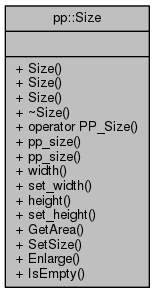Pepper_31_C++_interfaces
pp::Size Class Reference

A size of an object based on width and height. More...

`#include <size.h>`

Collaboration diagram for pp::Size:## Public Member Functions

Size ()
The default constructor. Initializes the width and height to 0. More...

Size (const PP_Size &s)

Size (int w, int h)

~Size ()
Destructor. More...

operator PP_Size ()

const PP_Size & pp_size () const

PP_Size & pp_size ()

int width () const

void set_width (int w)

int height () const

void set_height (int h)

int GetArea () const

void SetSize (int w, int h)

void Enlarge (int w, int h)

bool IsEmpty () const

## Detailed Description

A size of an object based on width and height.

Definition at line 18 of file size.h.

## Constructor & Destructor Documentation

 pp::Size::Size ( )
inline

The default constructor. Initializes the width and height to 0.

Definition at line 22 of file size.h.

 pp::Size::Size ( const PP_Size & s )
inline

A constructor accepting a pointer to a `PP_Size` and converting the `PP_Size` to a `Size`. This is an implicit conversion constructor.

Parameters
 [in] s A pointer to a `PP_Size`.

Definition at line 32 of file size.h.

References set_height(), and set_width().

 pp::Size::Size ( int w, int h )
inline

A constructor accepting two int values for width and height and converting them to a `Size`.

Parameters
 [in] w An int value representing a width. [in] h An int value representing a height.

Definition at line 43 of file size.h.

References set_height(), and set_width().

 pp::Size::~Size ( )
inline

Destructor.

Definition at line 50 of file size.h.

## Member Function Documentation

 void pp::Size::Enlarge ( int w, int h )
inline

Enlarge() enlarges the size of an object.

Parameters
 [in] w A width to add the current width. [in] h A height to add to the current height.

Definition at line 131 of file size.h.

References height(), set_height(), set_width(), and width().

 int pp::Size::GetArea ( ) const
inline

GetArea() determines the area (width * height).

Returns
The area.

Definition at line 114 of file size.h.

References height(), and width().

 int pp::Size::height ( ) const
inline

Getter function for returning the value of height.

Returns
The value of height for this `Size`.

Definition at line 96 of file size.h.

Referenced by Enlarge(), GetArea(), IsEmpty(), operator==(), and pp::Rect::set_size().

 bool pp::Size::IsEmpty ( ) const
inline

IsEmpty() determines if the size is zero.

Returns
true if the size is zero.

Definition at line 139 of file size.h.

References height(), and width().

 pp::Size::operator PP_Size ( )
inline

PP_Size() allows implicit conversion of a `Size` to a `PP_Size`.

Returns
A Size.

Definition at line 57 of file size.h.

 const PP_Size& pp::Size::pp_size ( ) const
inline

Getter function for returning the internal `PP_Size` struct.

Returns
A const reference to the internal `PP_Size` struct.

Definition at line 64 of file size.h.

 PP_Size& pp::Size::pp_size ( )
inline

Getter function for returning the internal `PP_Size` struct.

Returns
A mutable reference to the `PP_Size` struct.

Definition at line 71 of file size.h.

 void pp::Size::set_height ( int h )
inline

Setter function for setting the value of height.

Parameters
 [in] h A new height value.

Definition at line 103 of file size.h.

References PP_DCHECK.

Referenced by Enlarge(), SetSize(), and Size().

 void pp::Size::set_width ( int w )
inline

Setter function for setting the value of width.

Parameters
 [in] w A new width value.

Definition at line 85 of file size.h.

References PP_DCHECK.

Referenced by Enlarge(), SetSize(), and Size().

 void pp::Size::SetSize ( int w, int h )
inline

SetSize() sets the value of width and height.

Parameters
 [in] w A new width value. [in] h A new height value.

Definition at line 122 of file size.h.

References set_height(), and set_width().

 int pp::Size::width ( ) const
inline

Getter function for returning the value of width.

Returns
The value of width for this `Size`.

Definition at line 78 of file size.h.

Referenced by Enlarge(), GetArea(), IsEmpty(), operator==(), and pp::Rect::set_size().

The documentation for this class was generated from the following file: